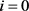# 非线性延迟波动方程的两类差分格式Two Difference Schemes for Nonlinear Wave Equations with Delay

DOI: 10.12677/PM.2020.105062, PDF, HTML, XML, 下载: 70  浏览: 143  国家自然科学基金支持

Abstract: This study is concerned with numerical solutions of delayed wave equations by explicit finite dif-ference methods. By using the discrete energy method, it is shown that both of them are temporally and spatially second-order convergent in maximum norm. Numerical findings confirm the accuracy and efficiency of the algorithms.

1. 引言

${u}_{tt}-{a}^{2}{u}_{xx}=f\left(u\left(x,t\right),u\left(x,t-s\right),x,t\right)$, ${b}_{1}, $0, (1a)

$u\left(x,t\right)=\varphi \left(x,t\right)$, ${b}_{1}, $-s\le t\le 0$, (1b)

$u\left({b}_{1},t\right)=\phi \left(t\right)$, $u\left({b}_{2},t\right)=\psi \left(t\right)$, $0\le t\le T$, (1c)

2. 差分格式

2.1. 记号

${\delta }_{t}^{2}{u}_{i}^{k}=\frac{1}{{h}_{t}^{2}}\left({u}_{i}^{k+1}-2{u}_{i}^{k}+{u}_{i}^{k-1}\right)$, ${\delta }_{\stackrel{^}{t}}{u}_{i}^{k}=\frac{1}{2{h}_{t}}\left({u}_{i}^{k+1}-{u}_{i}^{k-1}\right)$, ${\delta }_{t}{u}_{i}^{k-\frac{1}{2}}=\frac{1}{{h}_{t}}\left({u}_{i}^{k}-{u}_{i}^{k-1}\right)$,

${\delta }_{x}^{2}{u}_{i}^{k}=\frac{1}{{h}_{x}^{2}}\left({u}_{i+1}^{k}-2{u}_{i}^{k}+{u}_{i-1}^{k}\right)$, $\left(u,v\right)={h}_{x}\underset{i=1}{\overset{m-1}{\sum }}{u}_{i}{v}_{i}$, ${\left(u,v\right)}_{1}={h}_{x}\underset{i=1}{\overset{m-1}{\sum }}\left({\delta }_{x}{u}_{i-\frac{1}{2}}\right)\left({\delta }_{x}{v}_{i-\frac{1}{2}}\right)$,

$‖u‖=\sqrt{\left(u,u\right)}$, ${|u|}_{1}=\sqrt{{\left(u,u\right)}_{1}}$, ${|u|}_{1}=\sqrt{{\left(u,u\right)}_{1}}$.

2.2. 两类差分格式的建立

${u}_{tt}\left({x}_{i},{t}_{k}\right)={\delta }_{t}^{2}{U}_{i}^{k}-\frac{{h}_{t}^{2}}{12}\frac{{\partial }^{4}u\left({x}_{i},{\eta }_{ik}\right)}{\partial {t}^{4}}$, ${u}_{xx}\left({x}_{i},{t}_{k}\right)={\delta }_{x}^{2}{U}_{i}^{k}-\frac{{h}_{x}^{2}}{12}\frac{{\partial }^{4}u\left({\xi }_{ik},{t}_{k}\right)}{\partial {x}^{4}}$.

${\delta }_{t}^{2}{U}_{i}^{k}-{a}^{2}{\delta }_{x}^{2}{U}_{i}^{k}=f\left({U}_{i}^{k},{U}_{i}^{k-{n}_{1}},{x}_{i},{t}_{k}\right)+{\left({R}_{1}\right)}_{i}^{k}$, $0, $0, (2)

${\left({R}_{1}\right)}_{i}^{k}=\left({h}_{t}^{2}\frac{{\partial }^{4}u\left({x}_{i},{\eta }_{ik}\right)}{\partial {t}^{4}}-{a}^{2}{h}_{x}^{2}\frac{{\partial }^{4}u\left({\xi }_{ik},{t}_{k}\right)}{\partial {x}^{4}}\right)/12$, $0, $0. (3)

${u}_{i}^{k}$ 代替 ${U}_{i}^{k}$，略去小量项 ${\left({R}_{1}\right)}_{i}^{k}$，得到第一个差分格式

${\delta }_{t}^{2}{u}_{i}^{k}-{a}^{2}{\delta }_{x}^{2}{u}_{i}^{k}=f\left({u}_{i}^{k},{u}_{i}^{k-{n}_{1}},{x}_{i},{t}_{k}\right)$, $0, $0, (4a)

${u}_{i}^{k}=\varphi \left({x}_{i},{t}_{k}\right)$, $0, $-{n}_{1}\le k\le 0$, (4b)

${u}_{0}^{k}=\phi \left({t}_{k}\right)$, ${u}_{0}^{k}=\psi \left({t}_{k}\right)$, $0\le k\le n$. (4c)

$\begin{array}{c}{\delta }_{x}^{2}{U}_{i}^{k}=\frac{1}{{h}_{x}^{2}}\left({U}_{i+1}^{k}-2{U}_{i}^{k}+{U}_{i-1}^{k}\right)\\ =\frac{1}{{h}_{x}^{2}}\left[{U}_{i+1}^{k}-2\left(\frac{{U}_{i}^{k+1}+{U}_{i}^{k-1}}{2}-\frac{{h}_{t}^{2}}{2}\frac{{\partial }^{2}u\left({x}_{i},{\varsigma }_{ik}\right)}{\partial {t}^{2}}\right)+{U}_{i-1}^{k}\right]\\ =\frac{1}{{h}_{x}^{2}}\left({U}_{i+1}^{k}+{U}_{i-1}^{k}\right)-\frac{2}{{h}_{x}^{2}}{U}_{i}^{k}-\frac{1}{{h}_{x}^{2}}\left({U}_{i}^{k+1}+{U}_{i}^{k-1}\right)+\frac{2}{{h}_{x}^{2}}{U}_{i}^{k}+\frac{{h}_{t}^{2}}{{h}_{x}^{2}}\frac{{\partial }^{2}u\left({x}_{i},{\varsigma }_{ik}\right)}{\partial {t}^{2}}\\ ={\delta }_{x}^{2}{U}_{i}^{k}-\frac{{h}_{t}^{2}}{{h}_{x}^{2}}{\delta }_{t}^{2}{U}_{i}^{k}+\frac{{h}_{t}^{2}}{{h}_{x}^{2}}\frac{{\partial }^{2}u\left({x}_{i},{\varsigma }_{ik}\right)}{\partial {t}^{2}}\end{array}$. (5)

$\left(1+{r}^{2}\right){\delta }_{t}^{2}{U}_{i}^{k}-{\delta }_{x}^{2}{U}_{i}^{k}=f\left({U}_{i}^{k},{U}_{i}^{k-{n}_{1}},{x}_{i},{t}_{k}\right)+{\left({R}_{2}\right)}_{i}^{k}$, $0, $0, (6)

${\left({R}_{2}\right)}_{i}^{k}={\left({R}_{1}\right)}_{i}^{k}+{r}^{2}\frac{{\partial }^{2}u\left({x}_{i},{\varsigma }_{ik}\right)}{\partial {t}^{2}}$, $0, $0. (7)

$\left(1+{r}^{2}\right){\delta }_{t}^{2}{u}_{i}^{k}-{a}^{2}{\delta }_{x}^{2}{u}_{i}^{k}=f\left({u}_{i}^{k},{u}_{i}^{k-{n}_{1}},{x}_{i},{t}_{k}\right)$, $0, $0, (8a)

${u}_{i}^{k}=\varphi \left({x}_{i},{t}_{k}\right)$, $0, $-{n}_{1}\le k\le 0$, (8b)

${u}_{0}^{k}=\phi \left({t}_{k}\right)$, ${u}_{0}^{k}=\psi \left({t}_{k}\right)$, $0\le k\le n$. (8c)

2.3. 差分格式的收敛性分析

$\left(-{\delta }_{x}^{2}v,v\right)={‖{\delta }_{x}v‖}^{2}$, ${‖v‖}_{\infty }\le \frac{\sqrt{{b}_{2}-{b}_{1}}}{2}{|v|}_{1}$,

$‖v‖\le \frac{{b}_{2}-{b}_{1}}{\sqrt{6}}{|v|}_{1}$, ${h}_{x}{‖{\delta }_{x}v‖}^{2}\le 4{‖v‖}^{2}$.

${F}^{k+1}\le A+B{h}_{t}\underset{k=0}{\overset{K}{\sum }}{F}^{k}$, 则 $\underset{0\le k\le K+1}{\mathrm{max}}{F}^{k}\le A\mathrm{exp}\left(B\left(K+1\right){h}_{t}\right)$

$|{\left({R}_{1}\right)}_{i}^{k}|\le {c}_{1}\left({h}_{t}^{2}+{h}_{x}^{2}\right)$, $0\le i\le m$, $-{n}_{1}\le k\le n$, (9)

$|{\left({R}_{2}\right)}_{i}^{k}|\le {c}_{2}\left({h}_{t}^{2}+{h}_{x}^{2}+\frac{{h}_{t}^{2}}{{h}_{x}^{2}}\right)$, $0\le i\le m$, $-{n}_{1}\le k\le n$, (10)

$|f\left(u+{\epsilon }_{1},v+{\epsilon }_{2},x,t\right)-f\left(u,v,x,t\right)|\le {c}_{3}\left(|{\epsilon }_{1}|+|{\epsilon }_{2}|\right)$ (11)

${h}_{t}\le \sqrt{\frac{{\epsilon }_{0}}{{c}_{4}\left({b}_{2}-{b}_{1}\right)}}$, ${h}_{x}\le \sqrt{\frac{{\epsilon }_{0}}{{c}_{4}\left({b}_{2}-{b}_{1}\right)}}$,

${|{e}^{k}|}_{1}^{2}\le {c}_{4}{\left({h}_{t}^{2}+{h}_{x}^{2}\right)}^{2}$, $-{n}_{1}\le k\le n$, (12a)

${‖{e}^{k}‖}_{\infty }\le \frac{\sqrt{\left({b}_{2}-{b}_{1}\right){c}_{4}}}{2}\left({h}_{t}^{2}+{h}_{x}^{2}\right)$, $-{n}_{1}\le k\le n$, (12b)

${\delta }_{t}^{2}{e}_{i}^{k}-{a}^{2}{\delta }_{x}^{2}{e}_{i}^{k}=f\left({U}_{i}^{k},{U}_{i}^{k-{n}_{1}},{x}_{i},{t}_{k}\right)-f\left({u}_{i}^{k},{u}_{i}^{k-{n}_{1}},{x}_{i},{t}_{k}\right)+{\left({R}_{1}\right)}_{i}^{k}$, $1\le i\le m$, $1\le k\le n-1$, (13a)

${e}_{i}^{k}=0$, $0\le i\le m$, $-{n}_{1}\le k\le 0$, (13b)

${e}_{i}^{k}=0$, $i=0$$i=m$$0\le k\le n$. (13c)

$-{n}_{1}\le k\le 0$ 时， ${e}_{i}^{k}=0$，显然(12a)式与(12b)式成立。

${‖{e}^{k}‖}_{\infty }\le \frac{\sqrt{{b}_{2}-{b}_{1}}}{2}{|{e}^{k}|}_{1}\le {\epsilon }_{0}$, $1\le k\le l$.

$f\left({U}_{i}^{k},{U}_{i}^{k-{n}_{1}},{x}_{i},{t}_{k}\right)$$f\left({U}_{i}^{k}\right)$$f\left({u}_{i}^{k},{u}_{i}^{k-{n}_{1}},{x}_{i},{t}_{k}\right)$$f\left({u}_{i}^{k}\right)$。运用不等式 ${\left(a+b\right)}^{2}\le 2\left({a}^{2}+{b}^{2}\right)$ 和(11) 式可得

${‖f\left({U}^{k}\right)-f\left({u}^{k}\right)‖}^{2}\le 2{c}_{3}^{2}\left({‖{e}_{i}^{k}‖}^{\text{2}}+{‖{e}_{i}^{k-{n}_{1}}‖}^{\text{2}}\right)$, $1\le k\le l$. (14)

${H}^{k}={‖{\delta }_{t}{e}^{k+\frac{1}{2}}‖}^{2}+{a}^{2}\left({\delta }_{x}{e}_{i+\frac{1}{2}}^{k},{\delta }_{x}{e}_{i+\frac{1}{2}}^{k+1}\right)$。当 $r<1$ 时，对(13a)式两端同时与 $2{\delta }_{\stackrel{^}{t}}{e}^{k}$ 做內积，运用引理2.1得

$\frac{1}{{h}_{t}}\left({H}^{k}-{H}^{k-1}\right)=\left(\left[f\left({U}^{k}\right)-f\left({u}^{k}\right)\right],2{\delta }_{\stackrel{^}{t}}{e}^{k}\right)+\left({\left({R}_{1}\right)}_{i}^{k},2{\delta }_{\stackrel{^}{t}}{e}^{k}\right)$. (15)

$\begin{array}{c}{H}^{k}={‖{\delta }_{t}{e}^{k+\frac{1}{2}}‖}^{2}+{a}^{2}{|{e}^{k+\frac{1}{2}}|}_{1}^{2}-\left[{a}^{2}{|{e}^{k+\frac{1}{2}}|}_{1}^{2}-{a}^{2}\left({\delta }_{x}{e}_{i+\frac{1}{2}}^{k},{\delta }_{x}{e}_{i+\frac{1}{2}}^{k+1}\right)\right]\\ ={‖{\delta }_{t}{e}^{k+\frac{1}{2}}‖}^{2}+{a}^{2}{|{e}^{k+\frac{1}{2}}|}_{1}^{2}-\left({a}^{2}{|{e}^{k+1}-{e}^{k}|}_{1}^{2}\right)/4\\ =\left(1-{r}^{2}\right){‖{\delta }_{t}{e}^{k+\frac{1}{2}}‖}^{2}+{a}^{2}{|{e}^{k+\frac{1}{2}}|}_{1}^{2}+{r}^{2}{‖{\delta }_{t}{e}^{k+\frac{1}{2}}‖}^{2}-{a}^{2}{|{e}^{k+1}-{e}^{k}|}_{1}^{2}/4\\ \ge \left(1-{r}^{2}\right){‖{\delta }_{t}{e}^{k+\frac{1}{2}}‖}^{2}+{a}^{2}{|{e}^{k+\frac{1}{2}}|}_{1}^{2}\end{array}$. (16)

${‖{\delta }_{t}{e}^{k+\frac{1}{2}}‖}^{2}\le \frac{1}{1-{r}^{2}}{H}^{k}$, ${|{e}^{k+\frac{1}{2}}|}_{\text{1}}^{\text{2}}\le \frac{1}{{a}^{2}}{H}^{k}$. (17)

$\begin{array}{l}\left(\left[f\left({U}^{k}\right)-f\left({u}^{k}\right)\right],2{\delta }_{\stackrel{^}{t}}{e}^{k}\right)\\ \le \frac{{c}_{3}^{2}}{1-{r}^{2}}\left({‖{e}^{k}‖}^{2}+{‖{e}^{k-{n}_{1}}‖}^{2}\right)+\left(1-{r}^{2}\right)\left({‖{\delta }_{t}{e}^{k+\frac{1}{2}}‖}^{2}+{‖{\delta }_{t}{e}^{k-\frac{1}{2}}‖}^{2}\right)\\ \le \frac{{c}_{3}^{2}}{1-{r}^{2}}\left({‖{e}^{k}‖}^{2}+{‖{e}^{k-{n}_{1}}‖}^{2}\right)+\left({H}^{k}+{H}^{k-1}\right)\end{array}$. (18)

$\begin{array}{l}\left({\left({R}_{1}\right)}^{k},2{\delta }_{\stackrel{^}{t}}{e}^{k}\right)\\ \le \frac{\left(m-1\right){h}_{x}{c}_{1}^{2}}{2\left(1-{r}^{2}\right)}{\left({h}_{x}^{2}+{h}_{t}^{2}\right)}^{2}+\left(1-{r}^{2}\right)\left({‖{\delta }_{t}{e}_{i}^{k+\frac{1}{2}}‖}^{2}+{‖{\delta }_{t}{e}_{i}^{k-\frac{1}{2}}‖}^{2}\right)\\ \le \frac{\left({b}_{2}-{b}_{1}\right){c}_{1}^{2}}{2\left(1-{r}^{2}\right)}{\left({h}_{x}^{2}+{h}_{t}^{2}\right)}^{2}+\left({H}^{k}+{H}^{k-1}\right)\end{array}$. (19)

${\delta }_{x}{e}_{i+\frac{1}{2}}^{k+1}={\delta }_{x}{e}_{i+\frac{1}{2}}^{k+\frac{1}{2}}+\frac{{h}_{t}}{2}{\delta }_{x}{\delta }_{t}{e}_{i+\frac{1}{2}}^{k+\frac{1}{2}}={\delta }_{x}{e}_{i+\frac{1}{2}}^{k+\frac{1}{2}}+\frac{r}{2a}\left({\delta }_{t}{e}_{i+1}^{k+\frac{1}{2}}-{\delta }_{t}{e}_{i}^{k+\frac{1}{2}}\right)$, $0\le i\le m-1$. (20)

$\begin{array}{c}{\left({\delta }_{x}{e}_{i+\frac{1}{2}}^{k+1}\right)}^{2}\le 2{\left({\delta }_{x}{e}_{i+\frac{1}{2}}^{k+\frac{1}{2}}\right)}^{2}+\frac{{r}^{2}}{2{a}^{2}}{\left({\delta }_{t}{e}_{i+1}^{k+\frac{1}{2}}-{\delta }_{t}{e}_{i}^{k+\frac{1}{2}}\right)}^{2}\\ \le 2{\left({\delta }_{x}{e}_{i+\frac{1}{2}}^{k+\frac{1}{2}}\right)}^{2}+\frac{{r}^{2}}{{a}^{2}}\left[{\left({\delta }_{t}{e}_{i+1}^{k+\frac{1}{2}}\right)}^{2}+{\left({\delta }_{t}{e}_{i}^{k+\frac{1}{2}}\right)}^{2}\right]\end{array}$, $0\le i\le m-1$. (21)

${a}^{2}{|{e}^{k+1}|}_{1}^{2}\le 2{H}^{k}+\frac{2{r}^{2}}{1-{r}^{2}}{H}^{k}=\frac{2}{1-{r}^{2}}{H}^{k}$, $1\le k\le l$. (22)

$\begin{array}{l}\frac{1}{{h}_{t}}\left({H}^{k}-{H}^{k-1}\right)\\ \le 2\left({H}^{k}+{H}^{k-1}\right)+\frac{\left({b}_{2}-{b}_{1}\right){c}_{1}^{2}}{2\left(1-{r}^{2}\right)}{\left({h}_{x}^{2}+{h}_{t}^{2}\right)}^{2}+\frac{{c}_{3}^{2}}{1-{r}^{2}}\left({‖{e}^{k}‖}^{2}+{‖{e}^{k-{n}_{1}}‖}^{2}\right)\\ \le 2\left({H}^{k}+{H}^{k-1}\right)+\frac{\left({b}_{2}-{b}_{1}\right){c}_{1}^{2}}{2\left(1-{r}^{2}\right)}{\left({h}_{x}^{2}+{h}_{t}^{2}\right)}^{2}+\frac{{\left[\left({b}_{2}-{b}_{1}\right){c}_{3}\right]}^{2}}{6\left(1-{r}^{2}\right)}\left({|{e}^{k}|}_{1}^{2}+{|{e}^{k-{n}_{1}}|}_{1}^{2}\right)\\ \le 2\left({H}^{k}+{H}^{k-1}\right)+\frac{\left({b}_{2}-{b}_{1}\right){c}_{1}^{2}}{2\left(1-{r}^{2}\right)}{\left({h}_{x}^{2}+{h}_{t}^{2}\right)}^{2}+\frac{{\left(\left({b}_{2}-{b}_{1}\right){c}_{3}\right)}^{2}}{6{\left[\left(1-{r}^{2}\right)a\right]}^{2}}\left({H}^{k-1}+{H}^{k-{n}_{1}-1}\right)\end{array}$. (23)

${H}^{k}\le {h}_{t}\left\{4+\frac{2{\left[{c}_{3}\left({b}_{2}-{b}_{1}\right)\right]}^{2}}{3{\left[\left(1-{r}^{2}\right)a\right]}^{2}}\right\}\underset{p=0}{\overset{k}{\sum }}{H}^{p}+\frac{\left({b}_{2}-{b}_{1}\right){c}_{1}^{2}T}{2\left(1-{r}^{2}\right)}{\left({h}_{x}^{2}+{h}_{t}^{2}\right)}^{2}$. (24)

$\left\{\text{8}+\frac{4{\left[{c}_{3}\left({b}_{2}-{b}_{1}\right)\right]}^{2}}{3{\left[\left(1-{r}^{2}\right)a\right]}^{2}}\right\}{h}_{t}\le 1$ 时，在(24)式中运用引理2.2可推得

${H}^{k}\le \frac{{a}^{2}\left(1-{r}^{2}\right){c}_{4}}{2}\left({h}_{t}^{2}+{h}_{x}^{2}\right)$, $0\le k\le l$. (25)

${|{e}^{l+1}|}_{1}^{2}\le \frac{2}{{a}^{2}\left(1-{r}^{2}\right)}{H}^{l}\le {c}_{4}{\left({h}_{t}^{2}+{h}_{x}^{2}\right)}^{2}$, ${‖{e}^{l+1}‖}_{\infty }\le \frac{\sqrt{{b}_{2}-{b}_{1}}}{2}{|{e}^{l+1}|}_{1}\le \frac{\sqrt{\left({b}_{2}-{b}_{1}\right){c}_{4}}}{2}\left({h}_{t}^{2}+{h}_{x}^{2}\right)$.

${h}_{x}\le \sqrt{\frac{2{\epsilon }_{0}}{3\sqrt{{c}_{5}\left({b}_{2}-{b}_{1}\right)}}}$, ${h}_{t}\le \sqrt{\frac{2{\epsilon }_{0}}{3\sqrt{{c}_{5}\left({b}_{2}-{b}_{1}\right)}}}$, $\frac{{h}_{t}}{{h}_{x}}\le \sqrt{\frac{2{\epsilon }_{0}}{3\sqrt{{c}_{5}\left({b}_{2}-{b}_{1}\right)}}}$, (26)

${|{e}^{k}|}_{1}^{2}\le {c}_{5}{\left({h}_{t}^{2}+{h}_{x}^{2}+\frac{{h}_{t}^{2}}{{h}_{x}^{2}}\right)}^{2}$, $-{n}_{1}\le k\le n$, (27a)

${‖{e}^{k}‖}_{\infty }\le \frac{\sqrt{{b}_{2}-{b}_{1}}}{2}{|{e}^{k}|}_{\text{1}}\le \frac{\sqrt{{c}_{5}\left({b}_{2}-{b}_{1}\right)}}{2}\left({h}_{t}^{2}+{h}_{x}^{2}+\frac{{h}_{t}^{2}}{{h}_{x}^{2}}\right)$, $-{n}_{1}\le k\le n$, (27b)

$\left(1+{r}^{2}\right){\delta }_{t}^{2}{e}_{i}^{k}-{\delta }_{x}^{2}{e}_{i}^{k}=f\left({U}_{i}^{k}\right)-f\left({u}_{i}^{k}\right)+{\left({R}_{2}\right)}_{i}^{k}$, $0, $0 (28a)

${e}_{i}^{k}=0$, $0\le i\le m$, $-{n}_{1}\le k\le 0$, (28b)

${e}_{i}^{k}=0$,$i=m$, $0\le k\le n$. (28c)

$-{n}_{1}\le k\le 0$ 时， ${e}_{i}^{k}=0$，显然(27a)式与(27b)式成立。

${‖{e}^{k}‖}_{\infty }\le \frac{\sqrt{{b}_{2}-{b}_{1}}}{2}{|{e}^{k}|}_{1}\le {\epsilon }_{0}$, $1\le k\le l$.

${‖f\left({U}^{k}\right)-f\left({u}^{k}\right)‖}^{2}\le 2{c}_{3}^{2}\left({‖{e}_{i}^{k}‖}^{\text{2}}+{‖{e}_{i}^{k-{n}_{1}}‖}^{\text{2}}\right)$, $1\le k\le l$.(29)

${G}^{k}=\left(1+{r}^{2}\right){‖{\delta }_{t}{e}^{k+\frac{1}{2}}‖}^{2}+{a}^{2}\left({\delta }_{x}{e}_{i+\frac{1}{2}}^{k},{\delta }_{x}{e}_{i+\frac{1}{2}}^{k+1}\right)$，对(28a)式两端与 $2{\delta }_{\stackrel{^}{t}}{e}^{k}$ 做內积，运用离散的格林公式得

$\frac{1}{{h}_{t}}\left({G}^{k}-{G}^{k-1}\right)=\left(\left[f\left({U}^{k}\right)-f\left({u}^{k}\right)\right],2{\delta }_{\stackrel{^}{t}}{e}^{k}\right)+\left({\left({R}_{2}\right)}^{k},2{\delta }_{\stackrel{^}{t}}{e}^{k}\right)$. (30)

${G}^{k}\ge {‖{\delta }_{t}{e}^{k+\frac{1}{2}}‖}^{2}+{a}^{2}{|{e}^{k+\frac{1}{2}}|}_{1}^{2}$. (31)

${‖{\delta }_{t}{e}^{k+\frac{1}{2}}‖}^{2}\le {G}^{k}$, ${|{e}^{k+\frac{1}{2}}|}_{1}^{2}\le \frac{1}{{a}^{2}}{G}^{k}$. (32)

$\left(\left[f\left({U}^{k}\right)-f\left({u}^{k}\right)\right],2{\delta }_{\stackrel{^}{t}}{e}^{k}\right)\le {c}_{3}^{2}\left({‖{e}^{k}‖}^{2}+{‖{e}^{k-{n}_{1}}‖}^{2}\right)+\left({G}^{k}+{G}^{k-1}\right)$ (33)

$\left({\left({R}_{2}\right)}^{k},2{\delta }_{\stackrel{^}{t}}{u}^{k}\right)\le \frac{1}{2}{c}_{2}^{2}\left({b}_{2}-{b}_{1}\right){\left({h}_{x}^{2}+{h}_{t}^{2}+{\left(\frac{{h}_{t}}{{h}_{x}}\right)}^{2}\right)}^{2}+\left({G}^{k}+{G}^{k-1}\right)$. (34)

${a}^{2}{|{e}^{k+1}|}_{1}^{2}\le 2{G}^{k}+2{r}^{2}{G}^{k}=\left(2+2{r}^{2}\right){G}^{k}$, $1\le k\le l$. (35)

$\begin{array}{l}\frac{1}{{h}_{t}}\left({G}^{k}-{G}^{k-1}\right)\\ \le 2\left({G}^{k}+{G}^{k-1}\right)+\frac{1}{2}{c}_{2}^{2}\left({b}_{2}-{b}_{1}\right){\left({h}_{x}^{2}+{h}_{t}^{2}+{\left(\frac{{h}_{t}}{{h}_{x}}\right)}^{2}\right)}^{2}+{c}_{3}^{2}\left({‖{e}^{k}‖}^{2}+{‖{e}^{k-{n}_{1}}‖}^{2}\right)\\ \le 2\left({G}^{k}+{G}^{k-1}\right)+\frac{1}{2}{c}_{2}^{2}\left({b}_{2}-{b}_{1}\right){\left({h}_{x}^{2}+{h}_{t}^{2}+{\left(\frac{{h}_{t}}{{h}_{x}}\right)}^{2}\right)}^{2}+\frac{{\left({b}_{2}-{b}_{1}\right)}^{2}}{6}{c}_{3}^{2}\left({|{e}^{k}|}_{1}^{2}+{|{e}^{k-{n}_{1}}|}_{1}^{2}\right)\\ \le 2\left({G}^{k}+{G}^{k-1}\right)+\frac{1}{2}{c}_{2}^{2}\left({b}_{2}-{b}_{1}\right){\left({h}_{x}^{2}+{h}_{t}^{2}+{\left(\frac{{h}_{t}}{{h}_{x}}\right)}^{2}\right)}^{2}+\frac{{\left({b}_{2}-{b}_{1}\right)}^{2}\left(1+{r}^{2}\right)}{3{a}^{2}}{c}_{3}^{2}\left({G}^{k-1}+{G}^{k-{n}_{1}-1}\right)\end{array}$. (36)

${G}^{k}\le {h}_{t}\left\{4+\frac{\left(1+{r}^{2}\right){\left({b}_{2}-{b}_{1}\right)}^{2}}{3{a}^{2}}\right\}\underset{p=0}{\overset{k}{\sum }}{G}^{p}+\frac{\left({b}_{2}-{b}_{1}\right){c}_{2}^{2}}{2}{\left[{h}_{x}^{2}+{h}_{t}^{2}+{\left(\frac{{h}_{t}}{{h}_{x}}\right)}^{2}\right]}^{2}$. (37)

$\left(\text{8}+\frac{2\left(1+{r}^{2}\right){\left({b}_{2}-{b}_{1}\right)}^{2}}{3{a}^{2}}\right){h}_{t}<1$ 时，在(37)式运用引理2.2，我们有

${G}^{k}\le \frac{\left({b}_{2}-{b}_{1}\right){c}_{2}^{2}}{2}\mathrm{exp}\left\{\left[8+\frac{2\left(1+{r}^{2}\right){\left({b}_{2}-{b}_{1}\right)}^{2}}{3{a}^{2}}\right]T\right\}{\left[{h}_{x}^{2}+{h}_{t}^{2}+{\left(\frac{{h}_{t}}{{h}_{x}}\right)}^{2}\right]}^{2}$, $0\le k\le l$. (38)

${|{e}^{l+1}|}_{1}^{2}\le \frac{2\left(1+{r}^{2}\right)}{{a}^{2}}{G}^{l}\le {c}_{5}{\left({h}_{x}^{2}+{h}_{t}^{2}+{\left(\frac{{h}_{t}}{{h}_{x}}\right)}^{2}\right)}^{2}$, ${‖{e}^{l+1}‖}_{\infty }\le \frac{\sqrt{{b}_{2}-{b}_{1}}}{2}{|{e}^{l+1}|}_{\text{1}}\le \frac{\sqrt{\left({b}_{2}-{b}_{1}\right){c}_{5}}}{2}\left({h}_{x}^{2}+{h}_{t}^{2}+{\left(\frac{{h}_{t}}{{h}_{x}}\right)}^{2}\right)$.

3. 数值实验

${u}_{tt}-2{u}_{xx}=f\left(u\left(x,t\right),u\left(x,t-0.1\right),x,t\right)$, $\left(x,t\right)\in \left(1,2\right]×\left(0,1\right]$,

$u\left(x,t\right)=\mathrm{sin}\left(xt\right)$, $\left(x,t\right)\in \left(1,2\right]×\left[-0.1,0\right)$,

$u\left(1,t\right)=\mathrm{sin}t$, $u\left(2,t\right)=\mathrm{sin}\left(2t\right)$, $t\in \left[0,1\right]$,Table 1. FDM (4a)~(4c) maximum error for numerical solutionTable 2. FDM (8a)~(8c) maximum error for numerical solution

4. 结论

  熊君, 李俊民, 等. 一阶双曲型偏微分方程的模糊边界控制[J]. 数学物理学报, 2017, 37(3): 469-477.  张在斌, 孙志忠. 一类非线性延迟抛物偏微分方程的Crank-Nicolson型差分格式[J]. 数值计算与计算机应用, 2010, 31(2): 131-140.  池永日. 一类高精度非线性延迟抛物偏微分方程的紧差分格式[J]. 延边大学学报(自然科学版), 2010, 36(4): 287-290.  Xie, J., Deng, D. and Zheng, H. (2017) A Compact Difference Scheme for One-Dimensional Nonlinear Delay Reaction-Diffusion Equations with Variable Coefficient. IAENG International Journal of Applied Mathematics, 47, 14-19.  Xie, J., Deng, D. and Zheng, H. (2017) Fourth-Order Difference Solv-ers for Nonlinear Delayed Fractional Sub-Diffusion Equations with Variable Coefficients. International Journal of Modelling and Simulation, 37, 241-251. https://doi.org/10.1080/02286203.2017.1358133  张启峰, 张诚坚, 邓定文. 求解非线性时滞双曲型偏微分方程的紧致差分方法及Richardson外推算法[J]. 数值计算与计算机应用, 2013, 34(3): 167-176.  孙志忠. 偏微分方程数数值解法[M]. 北京: 科学出版社, 2012: 110-171.  Deng, D. (2018) Unified Compact ADI Methods for Solving Nonlinear Viscous and Nonviscous Wave Equations, Chinese Journal of Physics, 56, 2897-2915. https://doi.org/10.1016/j.cjph.2018.09.025